Notebook

# Maths

## Principal Components Analysis

The following chapter shows fundamentals of Principal Component Analysis. The code used can be found here.

In :
% run ./maths_functions.py


Often when dealing with multiple variables, not all of them are fully independent. For example let's assume a big group of people are asked about their preferences on various topics such as sports, favourite colours, movies, ideas. You would find out that maybe the people that like blue are also more likely to enjoy action movies. This is the main goal of PCA - to identify corellations between the variables and reduce the dimensionality of the problem.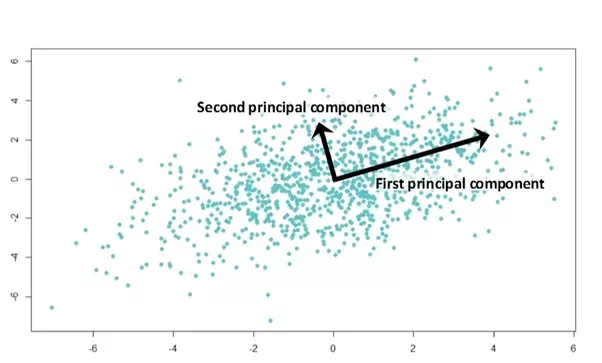The steps of getting the optimal principal components it the following:

1. Eigen-decomposition
2. Principal Components Selection
3. Dimensionality Reduction

#### Variable Definitions

So imagine we have $n$-entries of $m$ different standartized features. We represent each entry as a vector such as $\vec{x_n} = \begin{bmatrix} x_{n1} \ x_{n1} \ x_{n2} \ ... \ x_{nm} \end{bmatrix}$. The matrix of all enrty vectors is denoted as $X$. The mean value of each feature is $\mu_{m} = ({1}/{n}).\sum_{k=1}^{k=n} x_{km}$. The mean vector, $\vec{\mu}$, is a vector that contains all mean values from $\mu_1$ to $\mu_m$.

To summarize:

$n$ = entries,

$m$ = features of each entry,

$x_{nm}$ = value of m-th feature of n-th entry,

$X$ = matrix of entry vectors

$\mu$ = mean value of each feature,

$\vec{\mu}$ = vector of all mean values

The example dataset used deals with various features of both abnormal and normal ortopedic patients. For simplicity, the examples have been reduced to 10, however, keep in mind that in reality much larger datasets should be used.

In :
# Biomechanical Features of Orthopedic Patients
#  Lichman, M. (2013). UCI Machine Learning Repository
#  [http://archive.ics.uci.edu/ml].
#  University of California, School of Information and Computer Science

#  | pelvic    |  pelvic | lumbar lord- | sacral | pelvic  | degree_spondy-
#  | incidence |  tilt   | -osis angle  | slope  | radius  | -lolithesis
x1 = [80.112,    33.9424,  85.1016,       46.169,  125.594,  100.2921] #Abnormal
x2 = [95.480,    46.5501,  59.0000,       48.930,   96.684,   77.2830] #Abnormal
x3 = [74.095,    18.8237,  76.0322,       55.271,  128.406,   73.3882] #Abnormal
x4 = [87.679,    20.3656,  93.8224,       67.313,  120.945,   76.7306] #Abnormal
x5 = [48.260,    16.4175,  36.3291,       31.842,   94.882,   28.3438] #Abnormal
x6 = [38.505,    16.9643,  35.1128,       21.541,  127.633,    7.9867] #Normal
x7 = [54.921,    18.9684,  51.6015,       35.952,  125.847,    2.0016] #Normal
x8 = [44.362,     8.9454,  46.9021,       35.417,  129.221,    4.9942] #Normal
x9 = [48.319,    17.4521,  48.0000,       30.867,  128.980,   -0.9109] #Normal
x10= [45.702,    10.6599,  42.5778,       35.042,  130.178,   -3.3889] #Normal

X = [x1, x2, x3, x4, x5, x6, x7, x8, x9, x10]

n = 10

m = 6


The first step is to standartize/normalize the data in the range -1 to 1 with an average of 0 with the following formula:

$\sigma = \sqrt{ (\cfrac{\Sigma_{k=1}^{k=n}(x_k-\vec{\mu})^2}{n}) }$

$x'=\cfrac{x-\vec{\mu}}{\sigma}$

In :
def mean_feat (X):
'''
Returns mean vector of X relative to axis=0

Alternatively do
np.sum(X,0)/n
'''
return np.mean(X,0)
def std_dev (X, m, n):
'''
Returns the standard deviation

matrix form.
'''
mu = mean_feat (X)
feat_dev = X - mu
feat_dev_sqr = np.power(feat_dev,2)
feat_dev_sum = np.mean(feat_dev_sqr, 0)
feat_variance = np.sqrt (feat_dev_sum)
return feat_variance

In :
# Normalizing data
variance = std_dev(X,m,n)
mu = mean_feat(X)
X = np.divide((X-mu),variance)


### Eigen-decomposition

#### Covariance Matrix

The covariance matrix $\Sigma$ has size $m \times m$ where each cell $(i,j)$ contains the covariance of x_i with relation to x_j. The covariance is calculated using the following equation. The mean value has been normalized to 0 so it can be ignored:

$\Sigma_{ij} = \cfrac{1}{n} . \sum_{k=1}^{k=n} (x_{ki} - \mu_{i}). (x_{kj} - \mu_{k}) = \cfrac{1}{n} . (X - \vec{\mu_{i}})^T.(X - \vec{\mu_{k}}) = \cfrac{1}{n} . X^T.X$

Note that we are dividing by n, while sometimes it's better to divide by (n-1). To understand what is better to use in your case check out this link. In statistics, this number has to do with the degrees of freedom that we are dealing with.

In :
def covariance_matrix (X,n):
return np.dot(X.transpose(),X)/n

In :
cov = covariance_matrix(X,n)

In :
fig1, ax1= plt.subplots(1)
ax1.imshow(cov, aspect = 'auto', vmin=-1, vmax = 1)
for (j,i),label in np.ndenumerate(cov):
ax1.text(i,j,round(label,2),ha='center',va='center', color='white')
ax1.set_title('Covariance Matrix')
plt.show()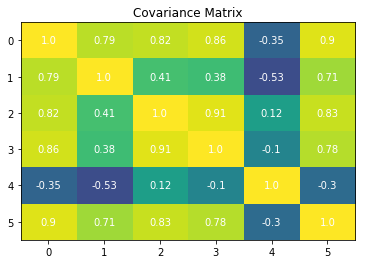A positive covariance means that the two features are postively related, while a negative covariance means that they are inversely ralated. A covariance equal to 1 means that the features are exactly the same - this is why the diagonal values are all one's.

#### Eigenvectors and Eigenvalues

For a matrix $A$, the eigenvector $\vec{v}$ and the eigenvalues $\lambda$ are the values that satisfy the equation $A.\vec{v}=\lambda.\vec{v}$. The eigenvectors represent the vectors that the covariance matrix scales up or down without changing direction. Eigenvalues are the values corresponding to the scaling factor of each eigenvector. In the examples below, the blue line shows the product vector Av. For the eigen case, the eigenvalue is 4.

In :
def plot_vector(v, ax):
ax.plot([0,v], [0,v])
return
def plot_vectors (v1, v2, title):
fig, ax = function_plot()
plot_vector(v1, ax)
plot_vector(v2, ax)
ax.set_title(title)
plt.show()
return

In :
v = [1,2]
A = np.array([[2, 2], [1, 3]])

plot_vectors(A.dot(v), v,  'Non-eigen product')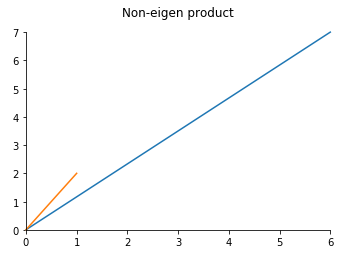In :
v = [1,1]

plot_vectors( A.dot(v), v, 'Eigen product')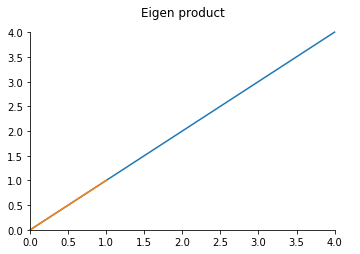$A.v=\lambda.v=\lambda.I.v$

$(A-\lambda.I)v=0$

$det(A-\lambda.I) = 0$

For an example matrix $A=\begin{bmatrix} a & b \\ c & d \end{bmatrix}$

$det(A-\lambda.I) = det(\begin{bmatrix} a-\lambda & b \\ c & d-\lambda \end{bmatrix})=0$

$(a-\lambda)(d-\lambda) - bc = 0$

From this equation the two roots for $\lambda$ are found. They can then be substituted into the initial equation. Finally, the eigenvector corresponding to each eigenvalue is found.

$\begin{bmatrix} a & b \\ c & d \end{bmatrix}. \begin{bmatrix} v_{11} \\ v_{12} \end{bmatrix} = \lambda_1 \begin{bmatrix} v_{11} \\ v_{12} \end{bmatrix}$

While this is not a hard equation to solve, it requires a lot more time and effort for matrices with more features such as the covariance matrix from before. Instead of solving manually, we can simply ask our Python to do it for us:

In :
eig_values, eig_vectors = np.linalg.eig(cov)
print (eig_values,'\n', eig_vectors)

[  4.07643550e+00   1.35082035e+00   4.01744801e-01   1.44166706e-01
9.84830682e-11   2.68326452e-02]
[[  4.88854790e-01   4.24142861e-02  -4.16914616e-02  -3.90461225e-01
-7.60646991e-01   1.62603909e-01]
[  3.73598593e-01   4.28391927e-01  -6.56864407e-01  -2.58017596e-01
4.14600061e-01  -8.34704061e-02]
[  4.28614842e-01  -4.13205749e-01   1.02711486e-02   1.62966670e-01
3.25579068e-05  -7.86693519e-01]
[  4.34293896e-01  -2.90969075e-01   4.81689313e-01  -3.80422045e-01
4.99522716e-01   3.16950058e-01]
[ -1.63805978e-01  -7.47849738e-01  -5.71772291e-01  -1.11562338e-01
-1.35036308e-05   2.72981068e-01]
[  4.71477271e-01   4.00663885e-04  -8.79620900e-02   7.72814162e-01
-8.28750263e-06   4.15607946e-01]]


### Principal Component Selection

Q: But what does this information mean to us?

A: The lower the eigenvalue, the less meaningful its eigenvector is.The larger the eigen value - the more relevant it is.

This allows us to drop the features/dimensions that we do not need.

In :
# A list of all eigenvalues and their corresponding eigenvectors
eigen_pairs = []
for i in range (m):
eigen_pairs.append([np.abs(eig_values[i]), eig_vectors[:,i]])
eigen_pairs.sort()

In :
for i in range (m):
print ( 'Value: %0.3e' % eigen_pairs[i],
' Vector:', np.array_str(eigen_pairs[i], precision=1))

Value: 9.848e-11  Vector: [ -7.6e-01   4.1e-01   3.3e-05   5.0e-01  -1.4e-05  -8.3e-06]
Value: 2.683e-02  Vector: [ 0.2 -0.1 -0.8  0.3  0.3  0.4]
Value: 1.442e-01  Vector: [-0.4 -0.3  0.2 -0.4 -0.1  0.8]
Value: 4.017e-01  Vector: [-0.  -0.7  0.   0.5 -0.6 -0.1]
Value: 1.351e+00  Vector: [  4.2e-02   4.3e-01  -4.1e-01  -2.9e-01  -7.5e-01   4.0e-04]
Value: 4.076e+00  Vector: [ 0.5  0.4  0.4  0.4 -0.2  0.5]


Q: How many features should we choose to keep?

A: To decide this, we will use a metric called expected variance which shows how much each principal component contributes to the information. The expected variance for each value is calculated as $\cfrac{ \lambda_i }{ \Sigma_{k=0}^{k=m} \lambda_k }$. The cumulative sum shows us the variance retained by the top-$n$ principal components. For this example, let's say we want to retain at least 95%.

In :
def pca_example(exp_variance, cumulative_variance):
fig, ax = function_plot()
ax.stem( np.linspace(1,m,m), exp_variance, label='Expected Variance')
plt.plot( np.linspace(1,m,m), cumulative_variance, label='Cumulative Variance')
ax.spines['bottom'].set_visible(False)
ax.set_ylim(0,100)
ax.set_xlim(1,m+0.1)
ax.set_xlabel('Principal Component')
ax.set_ylabel('Variance')
ax.axhline(95, color = 'red', label = '95% Variance Line')
for i in range (m):
ax.vlines(x=i+1, ymin=0, ymax= cumulative_variance[i],
color = 'b', linestyles='dotted')
ax.legend()
plt.show()
return

In :
exp_variance = 100 * eig_values / sum(eig_values)
cumulative_variance = np.cumsum(exp_variance)

pca_example(exp_variance, cumulative_variance)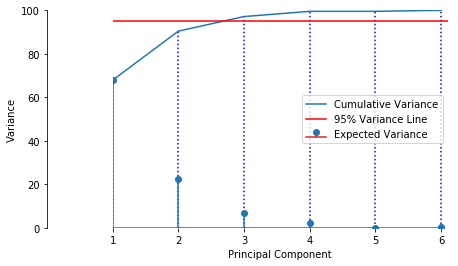As can be seen in the graph, the top-3 PC's retain more that 95% of the information, therefore we keep them and discard the other 3.

### Dimensionality Reduction

To reduce the dimensions, we multiply the initial examples with size ($n \times m$) by the selected k column eigenvectors with size ($m \times k$) to produce the examples in the new feature space with size ($n \times k$)

In :
top_k = eigen_pairs
top_k.reverse()
top_k = top_k [0:3]
W = np.hstack( top_k[i].reshape(6,1) for i in range (3))
Y= X.dot(W)


Since we chose the top-3 features, we can represent them in a 3-dimensional plot. Blue marks abnormal patients, red marks normal patients

In :
from mpl_toolkits.mplot3d import Axes3D
fig = plt.figure()
ax = Axes3D(fig)

for i in Y[0:5]:
ax.scatter( i, i, i, color = 'blue')
for i in Y[5:10]:
ax.scatter( i,  i, i, color = 'red')

zrange = Y[:,2].max() - Y[:,2].min()
yrange = Y[:,1].max() - Y[:,1].min()
xrange = Y[:,0].max() - Y[:,0].min()

ax.set_zlim (zmin = Y[:,2].min() - 0.25*zrange,
zmax = Y[:,2].max() + 0.25*zrange)
ax.set_ylim (ymin = Y[:,1].min() - 0.25*yrange,
ymax = Y[:,1].max() + 0.25*yrange)
ax.set_xlim (xmin = Y[:,0].min() - 0.25*xrange,
xmax = Y[:,0].max() + 0.25*xrange)

ax.set_xlabel('X Label')
ax.set_ylabel('Y Label')
ax.set_zlabel('Z Label')
plt.show()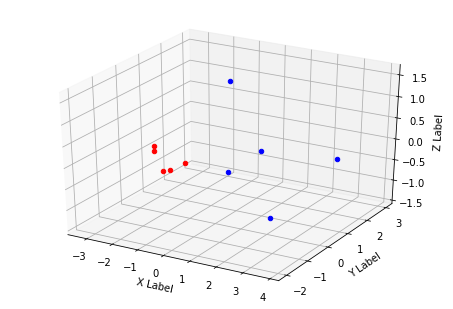As can be seen above, there is a clear divide between the two classes. The variance of the data is retained, while the dimensions are reduced from 6 to 3.